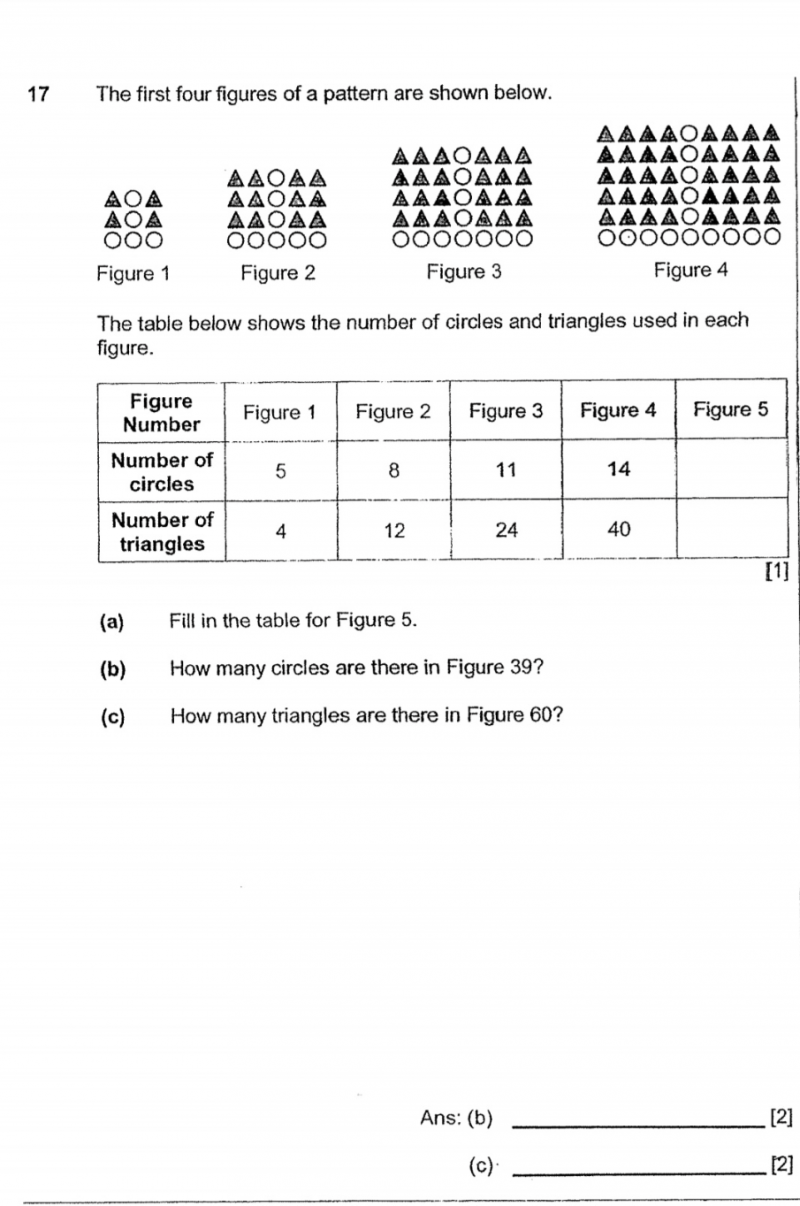# QuestionHi. Is there a specific way to solve this type pattern question? How to find the correct formula to get the answers ? Thanks

(a) 5,8,11,14  fig 5 = 14+3 = 17    nth term = 5+ 3(n-1) = 5 + 3n – 3 = 3n+2

4,12,24,40  = 4 (1,3,6,10…) = 4 [(n/2)(n+1)]  (1,3,6,10… is known as triangular number seq..) = 2n(n+1)

fig 5 = 4[(5/2)(6)] = 60  or  5×4 = 20  then 40+20 = 60

(b)  Fig 39 = 5 + 3(39-1) = 119 or  3(39) + 2 = 119

(c) Fig 60 = 4[(60/2 )(60+1)] = 7320 or 2(60)(60+1) = 7320

To answer the question of specific method…yes.

first sequence has a constant difference of 3

so you always fix the first number which is 5  then add 3(n-1) where 3(n-1) will be the generator of pattern as n varies…then simplify to become 3n+2

second sequence is a multiplier of a standard sequence called triangular number seq.(1,3,6,10…) and the nth term is (n/2)(n+1)  so  in this example it’s 4(n/2)(n+1) which can be simplified to 2n(n+1)